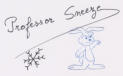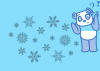Puzzle ! ... (8-10 years old)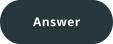N  O  P  W  R  S  T  I  E  F  G  N  U  V  W  D  O  P  Q
T  C  D  E  U  T  U  V  R  V  W  X  B  D  E  F  I  Q  R  S  N  J  K  L  E  F  G  H
When 3 letters follow each other in the alphabet correctly,
cross them out !

You will see the name of a machine
that produces renewable electricity,
which is good for the climate!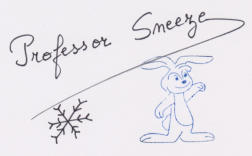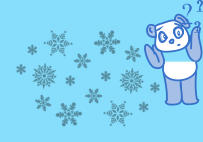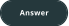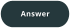N  O  P  W  R  S  T  I  E  F  G  N  U  V  W  D  O  P  Q
T  C  D  E  U  T  U  V  R  V  W  X  B  D  E  F  I  Q  R  S  N  J  K  L  E  F  G  H
When 3 letters follow each other in the alphabet correctly,
cross them out !

You will see the name of a machine
that produces renewable electricity,
which is good for the climate!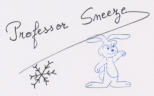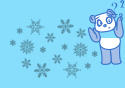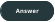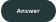N  O  P  W  R  S  T  I  E  F  G  N  U  V  W  D  O  P  Q
T  C  D  E  U  T  U  V  R  V  W  X  B  D  E  F  I  Q  R  S  N  J  K  L  E  F  G  H
When 3 letters follow each other in the alphabet correctly,
cross them out !